System ProgrammingLearn about Input/Output & File Handling in C++

# Learn about Input/Output & File Handling in C++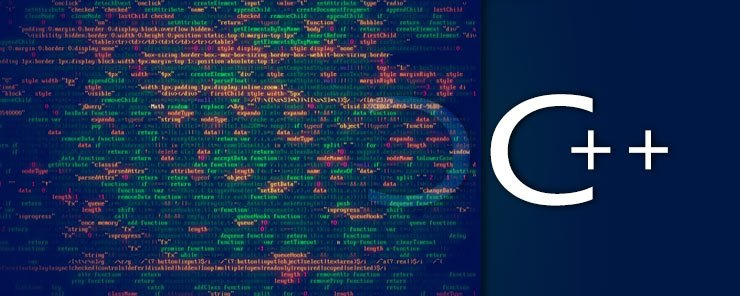In the previous article, we have learned how to run operating system commands from C++ programs on UNIX/Linux machines. In the same context, it is also essential for C++ code to have the capability to deal with files for input and output. When you hear a saying like “Everything in UNIX is a file”, you will realize why file handling is of utmost importance.
That will be the subject of our today’s article. Wish you nice reading.

Streams
The term “Stream” refers to flow of data. So far, we have been using two objects: cin and cout for input and output. I told you earlier in this series that cin and cout represent the standard input stream and standard output stream, respectively.

Introducing the ios Class
The ios class is the base of all stream classes. One of the main capabilities offered by this class is formatting. Formatting is done using Manipulators and Switches (flags). In this section, we are going to see how to use both manipulators and flags to customize the input/output format.

Manipulators
As their name implies, manipulators are formatting operators that manipulates (controls) the output formatting. Although manipulators also work with input stream, most of the time they are used to customize the way output is displayed. Manipulators are used directly with the stream extraction >> and insertion << operators.

The following are some of the commonly-used formatting manipulators, with their descriptions and usage examples.
endl
Inserts a newline character. This is equivalent to printing ‘\n’
Usage Example:

```#include <iostream>
using namespace std;
int main()
{
cout << "Hello World" << endl
<< "This is a formatted output";
return 0;
}```

This prints the following output: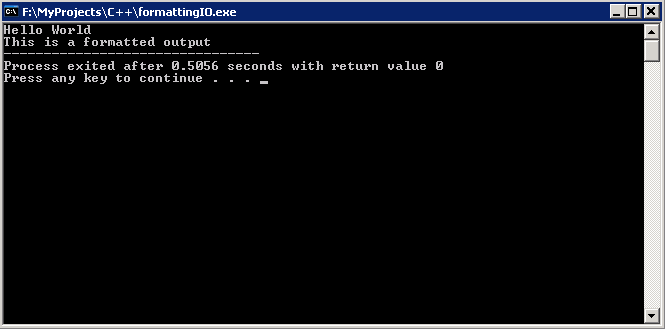hex
In output: the hex manipulator prints the hexadecimal equivalent of the number.
In Input: it treats the input as a hexadecimal number.

Usage Example
The following works like decimal to hexadecimal converter:

```#include <iostream>
#include <iomanip>
using namespace std;
int main()
{
long num;
cout << "Enter a positive integer: ";
cin >> num;
cout << "\nThe Hexadecimal equivalent of " << num << " is " << hex << num << endl;
return 0;
}```

This program works as follows: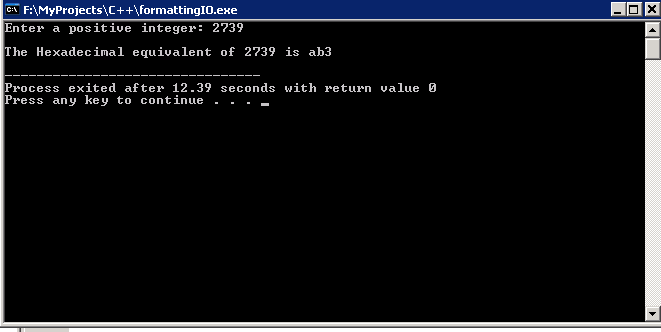Notice that the variable num has been printed twice. In the former, it was printed normally as it is (in decimal format). In the latter, its hexadecimal equivalent is printed instead. The reason is that the second num was preceded by the hex manipulator. This draws a very important conclusion: a manipulator affects only data following it, not that coming before it.

oct
Similar to hex, but accepts/prints the octal equivalent of the number.
dec
Converts to the decimal equivalent.

Usage Example

```#include <iostream>
#include <iomanip>
using namespace std;
int main()
{
long num;
cout << "Enter a hexadecimal number: ";
cin >> hex >> num;
cout << "\nOctal: " << oct << num << endl;
cout << "\nDecimal: " << dec << num << endl;
return 0;
}```

This program works as follows: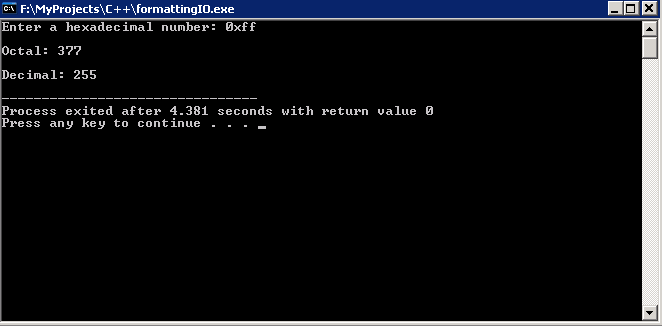setw()
The setw() manipulator function adjusts the field width in output.

Usage Example

```#include <iostream>
#include <iomanip>
using namespace std;
int main()
{
int num = 3490;
cout << "\nNumber: " << setw(10) << num << endl;
return 0;
}```

This will print the following output: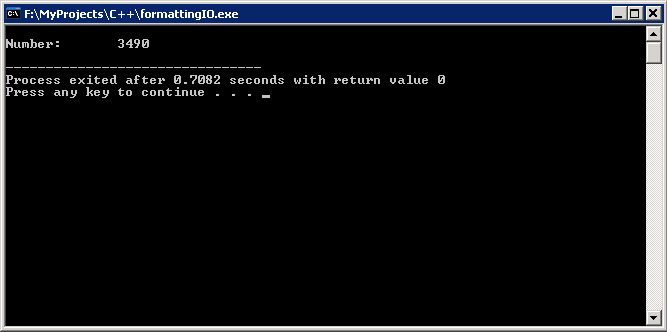setfill()
the setfill() function specifies the padding (filling) character to use. This function is meaningful only with setw().

Usage Example

```#include <iostream>
#include <iomanip>
using namespace std;
int main()
{
int num = 3490;
cout << "\nNumber: " << setw(10) << setfill('#') <<num << endl;
return 0;
}```

The number will be printed in 10-characters wide field, with blanks filled with #.

Flags
Format Flags are group of attributes defined in the ios class. These flags control the way input is interpreted, and output is printed.
Of the rich list of available format flags, we are going to discuss two commonly-used ones: left and right.
The left flag aligns output to left. Conversely, right aligns printed output to the right.

Example
Consider the following code:

```#include <iostream>
#include <iomanip>
using namespace std;
int main()
{
cout << endl;
cout.setf(ios::left); 	cout << setw(24) << "Item Name";
cout.setf(ios::right);  cout << setw(12) << "Price (USD)" << endl;
cout.unsetf(ios::right);
cout << endl;
cout.setf(ios::left); 	cout << setw(24) << "Smart TV 40\"";
cout.setf(ios::right);  cout << setw(12) << "3599.95" << endl;
cout.unsetf(ios::right);
cout.setf(ios::left); 	cout << setw(24) << "Tablet 10.1\"";
cout.setf(ios::right);  cout << setw(12) << "572.85" << endl;
return 0;
}```

When executed, this code should produce the following formatted output: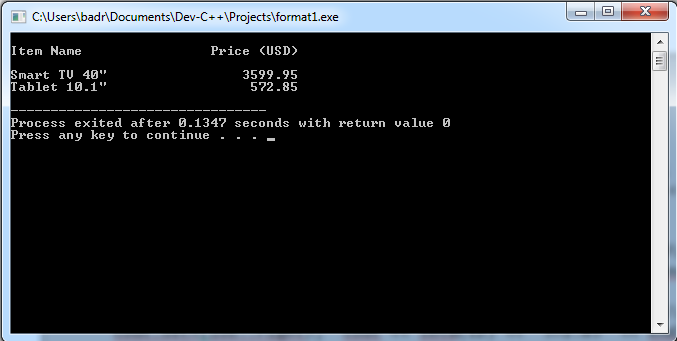The flags are set using the setf() function, and unset using the unsetf() function.

Summary

• A Stream refers to flow of data.
• Manipulators manipulate (control) the format of input and output.
• Manipulators are inserted directly into streams.
• Format Flags control the way input is interpreted, and output is printed. They are members of the ios

That was Part one in I/O and File Handling. See you in Part two.

### How Challenging Is B2B Marketing?

21,501Fans
4,217Followers
103,000Subscribers

### How Challenging Is B2B Marketing?

- Advertisement -